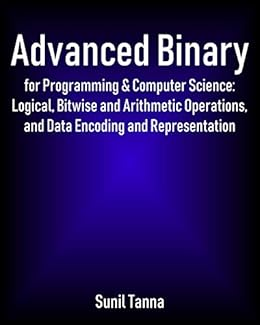#freebooks – Advanced Binary for Programming & Computer Science: Logical, Bitwise and Arithmetic Operations, and Data Encoding and Representation by Sunil Tannahttps://ift.tt/2PCTptL

Try Audible and Get Two Free AudiobooksThis book explains how the binary works and how it is used by computers to represent information including positive and negative integers, characters and real numbers. It explains the logical and bitwise operations used to manipulate information and perform arithmetic. We also briefly look at how computers store this information in memory and secondary storage, and how it can be transmitted between computers.

Topics covered include:

INTRODUCING NUMBER BASES AND BINARY

CONVERTING FROM BINARY TO DENARY AND VICE-VERSA

How to Convert a Binary Number to Denary

How to Convert a Denary Number to Binary

HOW COMPUTERS GROUP BINARY DIGITS

A Closer Look at Bytes

A Closer Look at Words

* Word alignment, word alignment and packing, byte ordering and endianness

BOOLEAN OPERATIONS AND LOGIC GATES

Fundamentals of Boolean Algebra

* NOT, AND, OR, XOR, NAND, NOR, NXOR

Combining Logic Gates

* NOT, AND, OR, XOR, NOR using NAND logic

Logical Versus Bitwise Operations

Using Bitwise Operations to Set, Clear, Flip or Test Bits

* Setting bits, inverting bits, clearing bits, testing bits

Subtracting Binary Integers

* The column subtraction methods of subtracting denary numbers and subtracting binary numbers, implementing binary subtraction using logic gates

SHIFT OPERATIONS

Left Shift

Right Shift

Circular Shifts

MULTIPLICATION AND DIVISION IN BINARY

Multiplication

* Multiplying by a power of 2, column multiplication, Russian peasant multiplication algorithm, multiplication in hardware

Division

* Dividing by a power of 2, denary long division, binary long division, algorithm for binary long division, division in hardware

REPRESENTING CHARACTERS AND STRINGS OF CHARACTERS

Representing Individual Characters

* ASCII, extended ASCIIs, BCDIC and other early character encodings, EBCDIC, Unicode

Representing Strings of Characters

* Terminated strings, length-prefixed strings, other string representations

REPRESENTING TEXT AND GRAPHICS ON SCREEN

Text Mode Displays

Bitmap Displays

PARITY CHECKING

What is a Parity Bit

Even and Odd Parity

Parity’s Use in RAID Storage Devices

Unused Parity Bits

SIGNED INTEGERS

Offset Binary

Signed Magnitude Representation

One’s Complement

Two’s Complement

Other Representations of Signed Numbers

* Base -2, signed-digit representation

REAL NUMBERS

Fixed Point Representation

Floating Point Representation

Rational Data Type

Logarithmic Number Systems

DENARY ENCODINGS AND DECIMAL DATA TYPES

Why Use Denary Representations of Real Numbers?

Binary Encodings of Denary

* Serial decimal, two-out-of-five, bi-quinary, character-based encodings of denary, binary-Coded Decimal (BCD), Chen-Ho Encoding, Densely Packed Decimal (DPD) and excess-3

Decimal Data Types

* Which numbers can be exactly represented in fixed and floating point?

* How inexact?

* Issues with inexact representation

* Decimal representation

DATA STRUCTURES

Structs

Arrays

Linked Lists and More Complex Structures

* Limitations of arrays, introducing linked lists, singly and doubly linked lists, more complex data structures

TYPES OF COMPUTER MEMORY

Magnetic-Core Memory and Core Rope Memory

RAM

* DRAM and SRAM

ROM

* Mask-programmed ROM, PROM, EPROM, EEPROM, Flash memory

SECONDARY STORAGE

Sequential Storage

* Punched tape, magnetic tape

Random Access Storage

* Magnetic disk, optical disk, solid state drives, flash memory and cloud Storage

MEASURING MEMORY AND STORAGE

DIGITAL COMMUNICATIONS

Serial Communication

Parallel Communication

MEASURING TRANSFER RATES

Baud